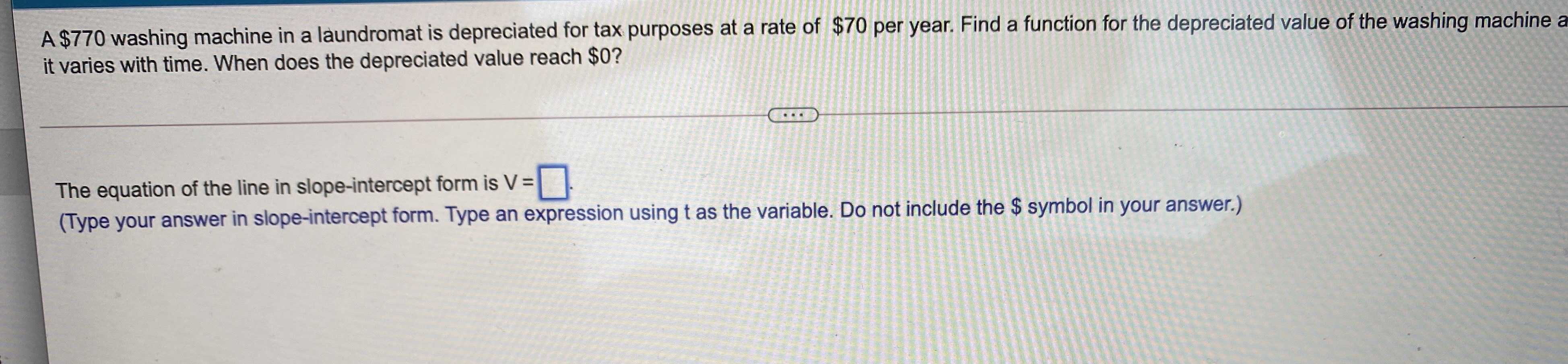### ¿Todavía tienes preguntas de matemáticas?

Pregunte a nuestros tutores expertos
Algebra
Pregunta$$\ 770$$ washing machine in a laundromat is depreciated for tax purposes at a rate of $$\ 70$$ per year. Find a function for the depreciated value of the washing machine a it varies with time. When does the depreciated value reach $$\ 0$$ ?

The equation of the line in slope-intercept form is $$V = \square$$ .

(Type your answer in slope-intercept form. Type an expression using $$t$$ as the variable. Do not include the $$\$$ symbol in your answer.)